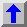Long Measure - Crazy World

1 league = 3 miles                                  = 5280 yards                                = 4828.032  m
1 mile = 8 furlongs                      = 1760 yards                                = 1609.344  m
1 furlong = 10 chains           =  220 yards                                =  201.168  m
1 chain = 4 rods   =   22 yards                                =   20.1168 m
1 rod    =    5.5 yards                              =    5.0292 m
1 fathom =    2 yards                                =    1.8288 m
1 yard = 3 feet                                    = 914.4 mm
1 foot = 12 inches                        = 304.8 mm
1 hand =  4 inches                        = 101.6 mm
1 inch   = 12 lines             =  25.4 mm
1 line  =  6 points =   2.11667 mm
1 point  =   0.35277 mm

1 league = 3 * 8 * 10 * 4 * 5.5 * 3 * 12 * 12 * 6 points = 13685760 points

Alternative

1 km = 1000 m                 = 1000000 mm
1 m = 10 dm         =    1000 mm
1 dm = 10 cm =     100 mm
1 cm =      10 mm